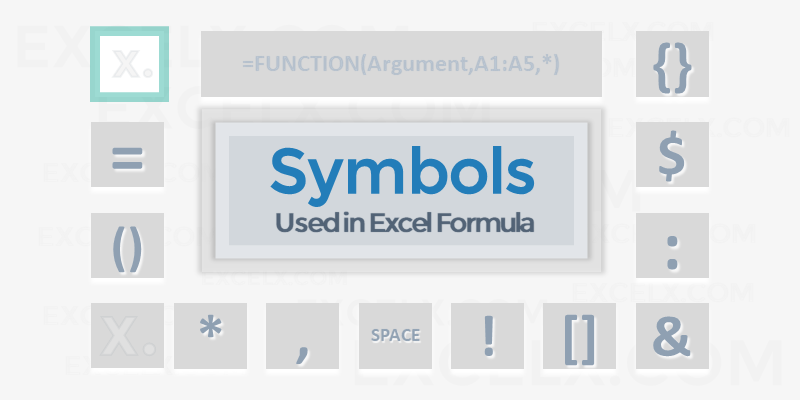Here are the important symbols used in Excel Formulas. Each of these special characters have used for different purpose in Excel. Let us see complete list of symbols used in Excel Formulas, its meaning and uses.

## Symbols used in Excel Formula

Following symbols are used in Excel Formula. They will perform different actions in Excel Formulas and Functions.

Symbol Name Description
= Equal to Every Excel Formula begins with Equal to symbol (=).

Example:=A1+A5

() Parentheses All Arguments of the Excel Functions specified between the Parentheses.

Example:=COUNTIF(A1:A5,5)

() Parentheses Expressions specified in the Parentheses will be evaluated first. Parentheses changes the order of the evaluation in Excel Formula.

Example: =25+(35*2)+5

* Asterisk Wild card operator to to denote all values in a List.

Example: =COUNTIF(A1:A5,”*“)

, Comma List of the Arguments of a Function Separated by Comma in Excel Formula.

Example: =COUNTIF(A1:A5,“>” &B1)

& Ampersand Concatenate Operator to connect two strings into one in Excel Formula.

Example: =”Total: “&SUM(B2:B25)

\$ Dollar Makes Cell Reference as Absolute in Excel Formula.

Example:=SUM(\$B\$2:\$B\$25)

! Exclamation Sheet Names and Table Names Followed by ! Symbol in Excel Formula.

Example: =SUM(Sheet2!B2:B25)

[] Square Brackets Uses to refer the Field Name of the Table (List Object) in Excel Formula.

Example:=SUM(Table1[Column1])

{} Curly Brackets Denote the Array formula in Excel.

Example: {=MAX(A1:A5-G1:G5)}

: Colon Creates references to all cells between two references.

Example: =SUM(B2:B25)

, Comma Union Operator will combine the multiple references into One.

Example: =SUM(A2:A25, B2:B25)

(space) Space Intersection Operator will create common reference of two references.

Example: =SUM(A2:A10 A5:A25)

1. Redjen May 31, 2022 at 12:37 pm - Reply

what is the symbol of average in excel?

• PNRao June 20, 2022 at 12:40 pm - Reply

You can use AVERAGE()Function to calculate Average in Excel. If you wants to show the Average Statistical Symbol (x-bar), You can insert from symbols. F7C2 is the Unicode Hexa character for X-bar symbol. Make sure that you have set the Symbol Font :MS Reference Sans Serif.

Excelx.com is a Excel Blog by PNRao to provide latest tips of Excel and help you to Quickly Learn and up-to date with Excel Concepts. We help you to know – how to do things in Excel with Clean and Easy Excel Tips, Shortcuts, Reference, Tutorials and New and Quick Tricks and Methods.

We Provide Free Online Tutorials, Formulas, Templates, Dashboards and Macros to excel in Microsoft Excel. We cover basic to advanced concepts and share creative ideas, Excel tips and Shortcuts with Related Example Files. You Can refer verity of examples covered on latest tools and techniques in Microsoft Excel Applications.